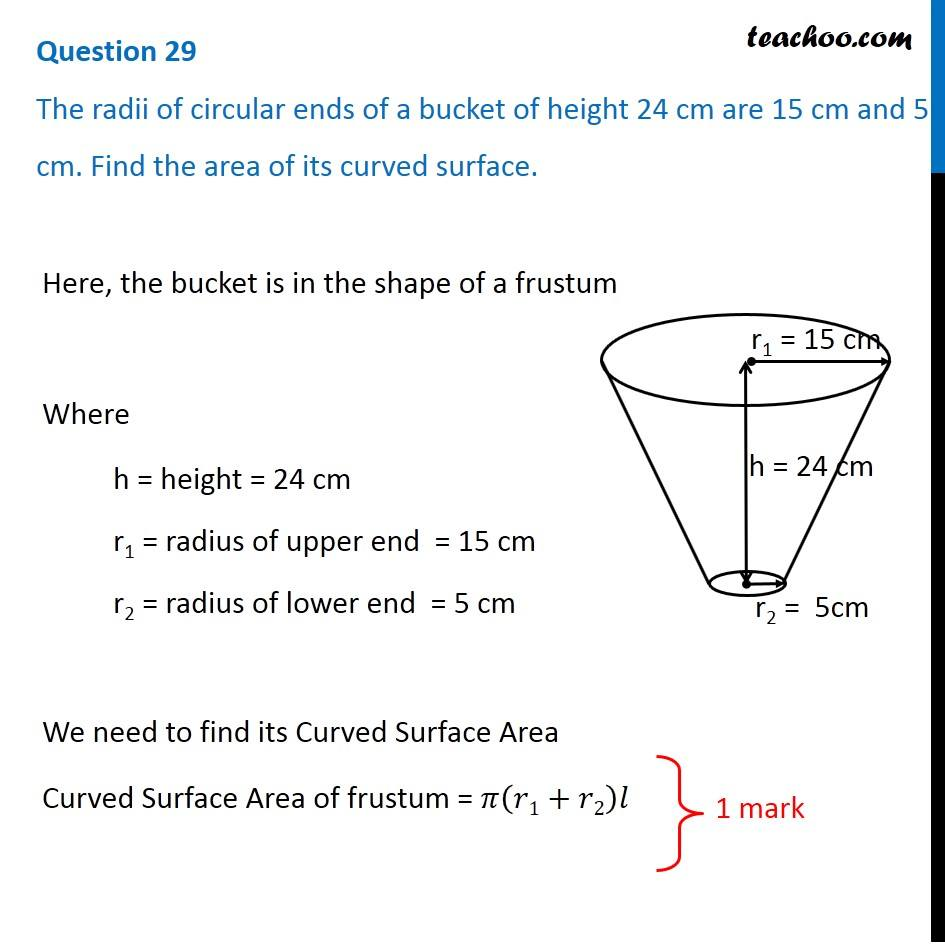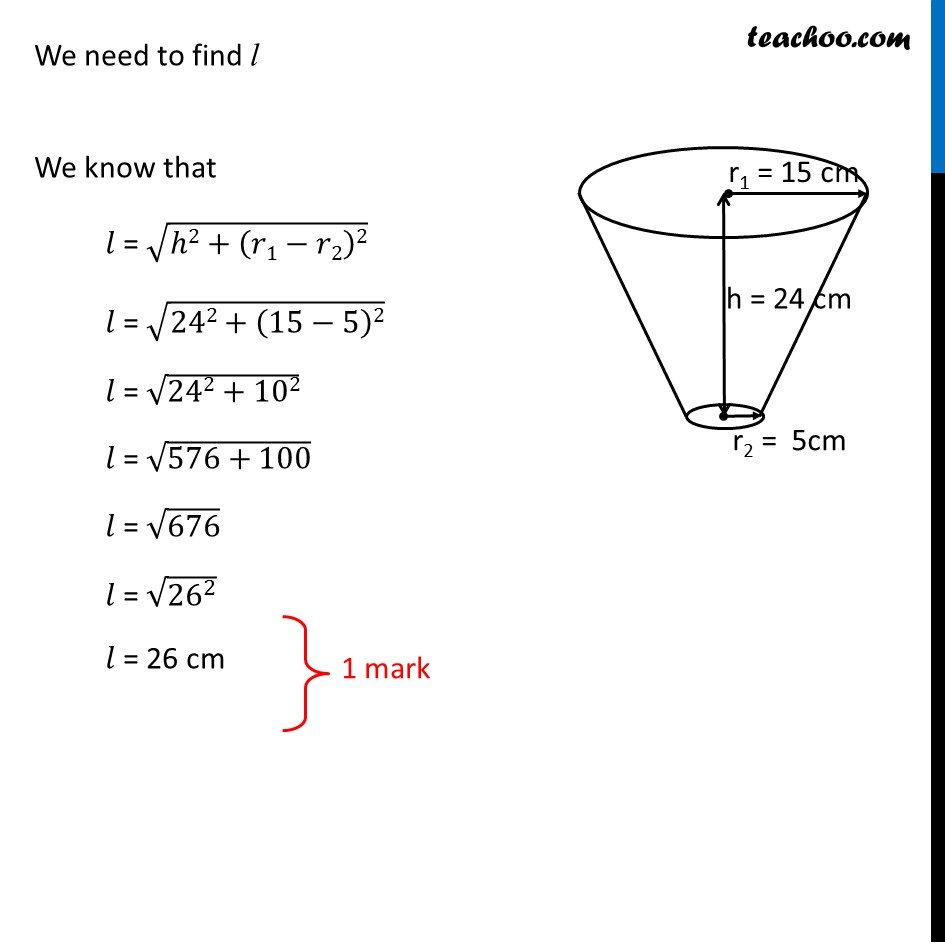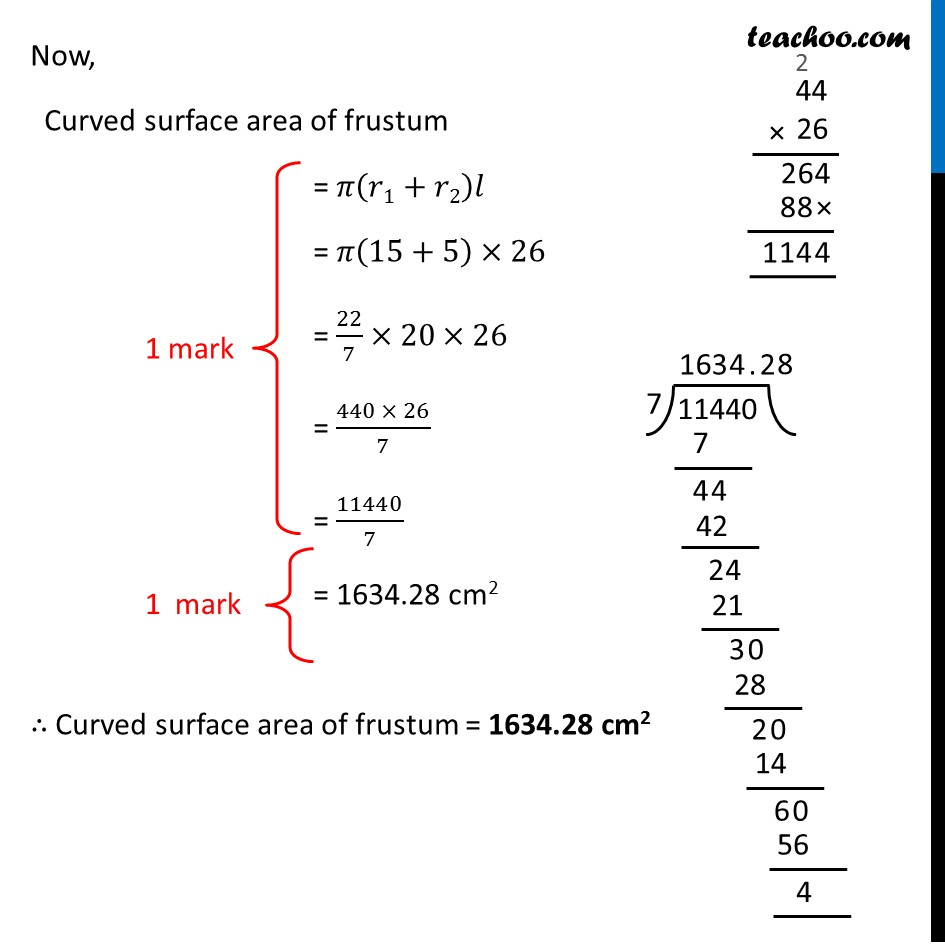CBSE Class 10 Sample Paper for 2019 Boards

Class 10
Solutions of Sample Papers for Class 10 Boards

Question 29

The radii of circular ends of a bucket of height 24 cm are 15 cm and 5 cm. Find the area of its curved surface.Learn in your speed, with individual attention - Teachoo Maths 1-on-1 Class

### Transcript

Question 29 The radii of circular ends of a bucket of height 24 cm are 15 cm and 5 cm. Find the area of its curved surface. Here, the bucket is in the shape of a frustum Where h = height = 16 cm r1 = radius of upper end = 20 cm r2 = radius of lower end = 8 cm We need to find its Curved Surface Area Curved Surface Area of frustum = 𝜋(𝑟1+𝑟2)𝑙 We need to find l We know that 𝑙 = √(ℎ2+(𝑟1−𝑟2)2) 𝑙 = √(242+(15−5)2) 𝑙 = √(242+102) 𝑙 = √(576+100) 𝑙 = √676 𝑙 = √(〖26〗^2 ) 𝑙 = 26 cm Now, Curved surface area of frustum = 𝜋(𝑟1+𝑟2)𝑙 = 𝜋(15+5)×26 = 22/7×20×26 = (440 × 26)/7 = 11440/7 = 1634.28 cm2 ∴ Curved surface area of frustum = 1634.28 cm2